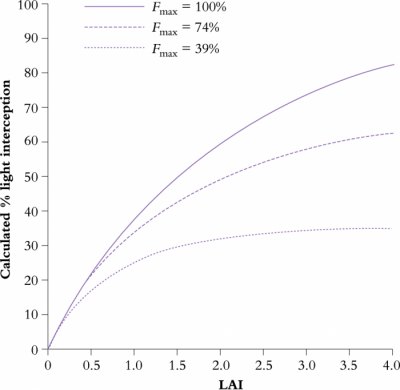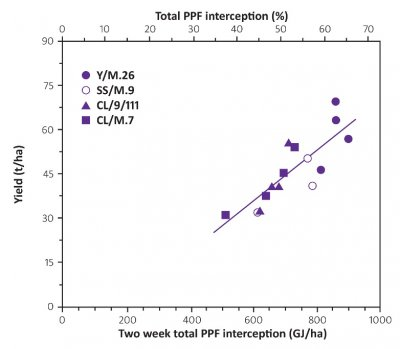# 12.4.1 - Light interception

An upper limit on dry matter production by crops is set by the amount of visible radiation (400–700 nm) intercepted (Monteith 1977). Horticultural crops, however, rarely achieve 100% interception of sunlight because physical access is required year round for routine management operations such as spraying, pruning and picking. This is especially so for perennial fruit crops and vines, but is also the case for many annual greenhouse and vegetable crops that are repeatedly hand harvested. With a closed agronomic canopy such as a ﬁeld of wheat, light interception is largely determined by LAI. With discontinuous canopies such as an apple orchard or a vineyard, a ﬁxed LAI can intercept different amounts of light depending upon the display in space of the discrete canopy units, namely individual trees or vines. (LAI for discontinuous canopies is calculated from the total area of leaves on the plant divided by the area allocated to the plant.)

Light interception of horticultural crops can be altered by changing spacing, tree height, tree shape and/or row orientation. An illustration of this is given in Table 12.1 using a simulation model of light interception (Palmer 1989). The model calculated light interception over a whole day and allowed for change in incident radiation, sun angle (both elevation and azimuth) and sky light. The model was used to calculate light interception under sunny conditions by apple trees arranged in continuous rows, of truncated conical cross-section, in midsummer at Riwaka, Nelson, New Zealand. For a constant LAI, light interception increased by increasing hedge height, closer row spacings and planting in a north–south rather than an east–west orientation (Table 12.1). Light interception varied by a factor of two, from 27% by short wide-spaced hedges in east–west (EW) rows to 55% by tall close spacings in north–south (NS) rows. Differences due to row orientation will be smaller in cloudy regions where more of the incoming light is diffuse.

## 12.4-Ch-Tab-12.1.png

In Table 12.1, the density of leaves within the tree volume changes with height and row spacing to maintain a constant LAI. Differences in light interception would be much larger if leaf area density rather than LAI was maintained at a constant. Nevertheless, data given in Table 12.1 for discontinuous canopies show that the relationship between LAI and light interception depends on the physical arrangement of foliage in space. Calculations for Table 12.1 assume an extinction coefﬁcient appropriate for apples. Light interception would have to be recalculated for other crops such as peach and cherry that have lower extinction coefﬁcients (Flore 1994).

As discussed in Section 12.3, the relationship between light interception ($F$) and LAI of a continuous canopy follows the Beer–Lambert Law, and can be approximated by Equation 12.5. Again, $k$ (the extinction coefﬁcient) is dependent upon leaf angle distribution, leaf transmission to sunlight and whether the foliage is random, regular or clumped. $k$ varies between about 0.3 for erect foliage and 1.0 for canopies comprising horizontally disposed leaves. In general terms,

$F = 1 - e^{–k\mathrm{LAI}} \tag{12.5}$

Unlike closed canopy forests or a continuous crop cover, sunlight interception by discontinuous canopies consists of two parts, a fraction determined by the overall form and extent of the canopy ($F_\mathrm{max}$) and a leaf area part that determines how close a particular orchard approaches the maximum interception attainable. $F_\mathrm{max}$ would be reached if LAI was inﬁnite. Accordingly, Equation 12.5 can be rewritten for discontinuous canopies as Equation 12.6, where $L' = \mathrm{LAI}/F_\mathrm{max}$ (Jackson and Palmer 1979).

$F = F_\mathrm{max} (1 - e^{–kL'}) \tag{12.6}$

This relationship for a discontinuous canopy contrasts with that for the continuous canopy (Equation 12.5) where an inﬁnite LAI would cause complete exclusion of light. When light interception by two of the orchards in Table 12.1 is plotted as a function of LAI (Figure 12.29) and compared with a continuous canopy with the same extinction coefﬁcient, light interception by either orchard approached an asymptote well short of inﬁnite LAI.

## 12.4-Ch-Fig-12.29.pngFigure 12.29 Calculated interception of sunlight as a function of LAI and canopy form. Light interception is calculated for a sunny day on 31 January at Riwaka, New Zealand (Lat. 41°6'S), for apply orchards arranged in continuous hedgerows. (Original data courtesy J.W. Palmer)

Orchards were either a continuous canopy ($F_\mathrm{max}$ = 100%), hedgerows 2 m tall, with 3 m row spacing, 1.5 m thick at the base and 0.5 m at the top ($F_\mathrm{max}$ = 74%) or hedgerows 1 m tall, with 5 m row spacing, 1.5 m thick at the base and 0.5 m at the top ($F_\mathrm{max}$ = 39%). Note that light interception depended on both LAI and $F_\mathrm{max}$, so that the same light interception can be achieved with a very different LAI or the same LAI can be rearranged to intercept a different amount of sunlight.

Light interception by discontinuous orchard canopies can, therefore, be increased by increasing LAI, but a far more effective strategy is to increase canopy extent. Put another way, it is better to use orchard design to increase $F_\mathrm{max}$ and thereby intercept sunlight that would otherwise fall into clear alleyways, rather than intercept sunlight that had a good chance of being absorbed by other foliage. Knowing the physical dimensions of the canopy, $F_\mathrm{max}$ can be calculated from a mathematical model. $F_\mathrm{max}$ complex shapes can be determined from CAD graphics.

## 12.4-Ch-Fig-12.30.pngFigure 12.30 Fruit crop yields as a function of light interception for apple trees with different rootstocks. (Based on Wünsche et al. 1996)

As for annual crops and forest canopies, there is also a linear relationship between biomass production and canopy light interception for horticultural crops, even though the horticultural yield is measured as fruit weight rather than biomass (Figure 12.30. Thus in all managed cropping systems, a universal relationship between canopy light interception and yield or biomass production and the LAI, whether based on continuous or discontinuous canopies, underpins this relationship through determining the extent of light interception by the canopy.